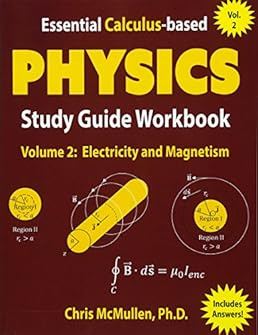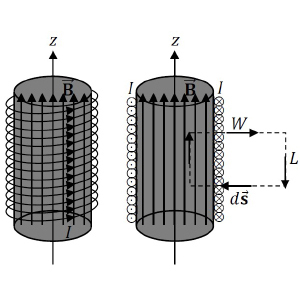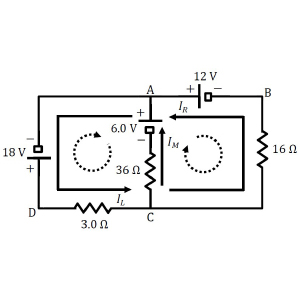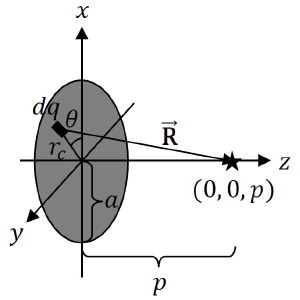# Essential Calculus-based Physics Study Guide Workbook: Electricity and Magnetism (Learn Physics with Calculus Step-by-Step) (Volume 2)Price: \$21.99
(as of Apr 15,2022 05:11:13 UTC – Details)

From the Publisher### Electricity and Magnetism (Physics with Calculus)

Chris McMullen, Ph.D.

Learn physics from a university instructor with over 20 years of experience teaching calculus-based (and trig-based) physics in California, Louisiana, Oklahoma, and Pennsylvania.

Clear explanations; communication is vital toward learning physics.Emphasis on understanding the connection between the math and the concepts.Explaining key steps in the math.A dedicated chapter reviews integration techniques and coordinate systems.Hints at the back (in addition to an answer key) help walk you through the solution. The hints address common questions. Designed to help students.

Each chapter concisely reviews key information.Relevant formulas are provided and explained.Handy charts help you quickly understand the symbols and units.Step-by-step examples serve as a handy guide.Stuck on a solution? Check the hints section at the back of the book.The answer to each problem is given at the bottom of the page for easy access.Lenz’s law is broken down into four simple steps.

Topics covered in Volume 2: Coulomb’s law, electric field, electrostatics electric field integrals, Gauss’s law, electric potential circuits with capacitors, circuits with resistors, Ohm’s law the resistor cube, Kirchhoff’s rules, resistivity, RC circuits magnetic field, magnetic force, magnets, right-hand rules Ampere’s law, the law of Biot-Savart, Faraday’s law, Lenz’s law, inductance AC circuits Maxwell’s equations### Both classic and new problems are included.

Some classic problems/examples include:

superposition of electric fieldselectric field map for an electric dipoleGauss’s law and electric field integrals for standard geometriesequivalent capacitance, equivalent resistance, and Kirchhoff’s rulesthe three variations of the resistor cubeAmpere’s law and the law of Biot-Savart for standard geometriesMaxwell’s equations### This book includes problems that allow students to apply their calculus skills.

electric field integralsGauss’s lawelectric potential integralsRC circuitsmagnetic field integralsAmpere’s law

ISBN
1941691153 194169117X 1941691145 1941691161 1941691110 1941691196

Description
Physics with calculus, Volume 1 (motion), covered in detail (motion, Newton’s laws, circular motion, work/energy, satellites, center of mass, collisions, rotation). Over 100 fully solved examples of calculus-based physics problems. These are actually the solutions to the problems from Essential Calculus-based Physics Study Guide Workbook. Trig-based physics, Volume 1 (motion). It’s basically the calculus-based physics book without any of the calculus. Over 100 fully solved examples of trig-based physics problems. These are actually the solutions to the problems from Essential Trig-based Physics Study Guide Workbook. Physics with calculus, Volume 2 (electricity and magnetism), covered in detail. Physics with calculus, Volume 3 (waves, fluids, sound, heat, and light), covered in detail.

Number of pages
over 400 nearly 300 over 300 over 200 over 500 over 400

ISBN
1941691153 1463644086 1941691285 1453772804

Description
Essential skills from physics with calculus. Volume 1 covers motion. In-depth coverage of calculus-based physics. There is currently just one volume of the “Advanced Introduction,” whereas there are 3 volumes of “Essential Calculus-based Physics.” Modern physics covers special relativity, blackbody radiation, the Compton effect, the photoelectric effect, Bohr’s model, Heisenberg’s uncertainty principle, and Schrodinger’s equation. Detailed and in-depth coverage of thermal physics across undergraduate physics.

Number of pages
over 400 over 500 nearly 300 over 300

Publisher ‏ : ‎ Zishka Publishing (March 4, 2017)
Language ‏ : ‎ English
Paperback ‏ : ‎ 572 pages
ISBN-10 ‏ : ‎ 1941691110
ISBN-13 ‏ : ‎ 978-1941691113
Item Weight ‏ : ‎ 2.89 pounds
Dimensions ‏ : ‎ 8.5 x 1.29 x 11 inches